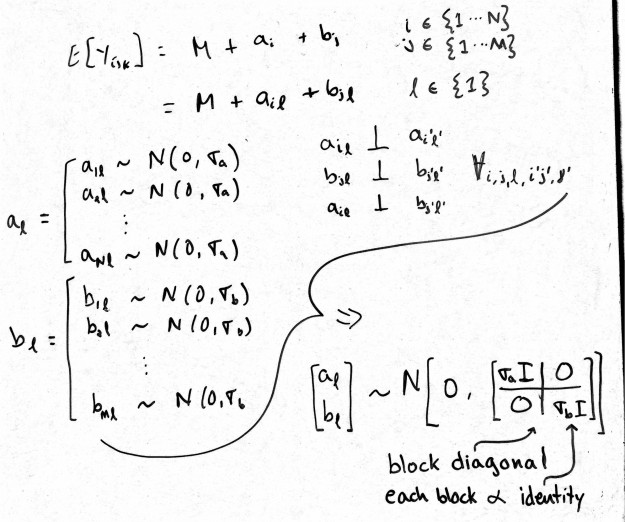# Nested vs. Non-nested (crossed) Random Effects in R

The R script below illustrates the nested versus non-nested (crossed) random effects functionality in the R packages lme4 and nlme. Note that crossed random effects are difficult to specify in the nlme framework. Thus, I've included a back-of-the-envelope (literally a scanned image of my scribble) interpretation of the 'trick' to specifying crossed random effects for nlme functions (i.e., nlme and lme).


## This script illustrates the nested versus non-nested
## random effects functionality in the R packages lme4 (lmer)
## and nlme (lme).

library("lme4")
library("nlme")
data("Oxide")
Oxide <- as.data.frame(Oxide)

## In the Oxide data, Site is nested in Wafer, which
## is nested in Lot. But, the latter appear crossed:
xtabs(~ Lot + Wafer, Oxide)

## So, create a variable that identifies unique Wafers
Oxide$LotWafer <- with(Oxide, interaction(Lot,Wafer)) ## For continuous response 'Thickness', ## fit nested model E[y_{ijk}] = a + b_i + g_{ij} ## for Lot i = 1:8 and Wafer j = 1:3 and Site k = 1:3 ## where b_i ~ N(0, \sigma_1) ## g_{ij} ~ N(0, \sigma_2) ## and b_i is independent of g_{ij} ## The following four models are identical: ## lme4 lmer(Thickness ~ (1 | Lot/Wafer), data=Oxide) lmer(Thickness ~ (1 | Lot) + (1 | LotWafer), data=Oxide) ## Note: the equivalence of the above formulations makes ## clear that the intercept indexed by Wafer within Lot ## has the same variance across Lots. ## nlme lme(Thickness ~ 1, random= ~1 | Lot/Wafer, data=Oxide) lme(Thickness ~ 1, random=list(~1|Lot, ~1|Wafer), data=Oxide) ## Note: the second formulation illustrates that lme assumes ## nesting in the order that grouping factors are listed. I ## think that this was a poor implementation decision, and ## that the latter should indicate non-nested grouping. ## Fit non-nested model E[y_{ijk}] = a + b_i + g_j ## for Lot i = 1:8 and Wafer j = 1:3 and Site k = 1:3 ## where b_i ~ N(0, \sigma_1) ## g_j ~ N(0, \sigma_2) ## and b_i is independent of g_j ## lme4 lmer(Thickness ~ (1 | Lot) + (1 | Wafer), data=Oxide) lmer(Thickness ~ (1 | Wafer) + (1 | Lot), data=Oxide) ## nlme: There is no 'easy' way to do this with the nlme package, ## and I couldn't get this to work at all with the nlme function. ## This is a trick that gives a random slope for each level of the ## grouping variables, which are indexed by the levels of ## a dummy grouping variable with only one group. We also ## specify, for each grouping factor, that covariance ## matrix is proportional to the identity matrix. Oxide$Dummy <- factor(1)
Oxide <- groupedData(Thickness ~ 1 | Dummy, Oxide)
lme(Thickness ~ 1, data=Oxide,
random=pdBlocked(list(pdIdent(~ 0 + Lot),
pdIdent(~ 0 + Wafer))))


The image below is my interpretation of the nlme (lme) trick for non-nested (crossed) random effects. The idea is to assign a random slope (no intercept) to each level of the grouping factors, which are each indexed by the levels of a dummy variable with that has exactly one level. The pdIdent function ensures that these random effects are uncorrelated and common variance. The pdBlocked function specifies that the random effects are also independent across the two grouping factors.